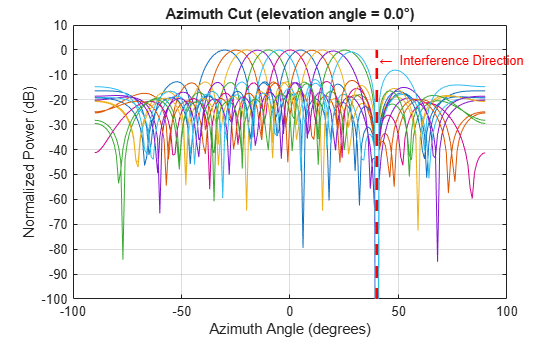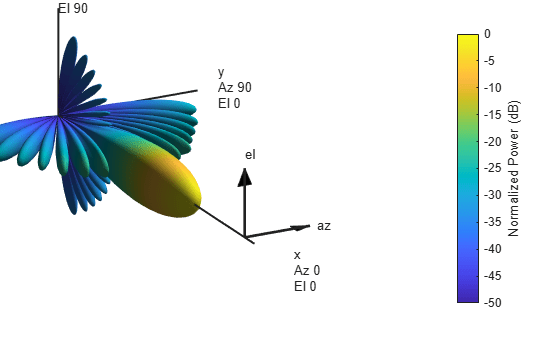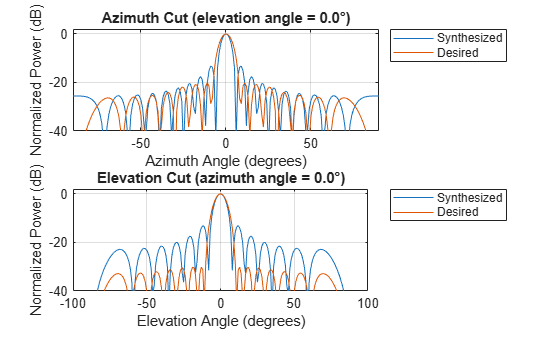## Array Pattern Synthesis

This example shows how to use Phased Array System Toolbox™ to solve some array synthesis problems.

In phased array design applications, it is often necessary to find a way to taper element responses so that the resulting array pattern satisfies certain performance criteria. Typical performance criteria include the mainlobe location, null location(s) and sidelobe levels.

### Interference Removal Using Sidelobe Canceller

A common requirement when synthesizing beam patterns is pointing a null towards a given arrival direction. This helps suppress interference from that direction and improves the signal-to-interference ratio. The interference is not always malicious- an airport radar system may need to suppress interference from a nearby radio station. In this case, the position of the radio station is known and a sidelobe cancellation algorithm can be used to remove the interference.

Sidelobe cancellation is useful for suppressing interference that enters through the array's sidelobes. In this case, because the interference direction is known, the algorithm is simple. Form a beam that points towards the interference direction, then scale the beam weights and subtract scaled weights from the weights for the beam patterns that point towards any other look direction. This process always places a strong null in the interference direction.

The following example shows how to design the weights of the radar so that it scans between -30 and 30 degrees yet always keeps a null at 40 degrees. Assume that the radar uses a 10-element ULA that is parallel to the ground and that the known radio interference arrives from 40 degrees azimuth.

```c = 3e8; % signal propagation speed fc = 1e9; % signal carrier frequency lambda = c/fc; % wavelength thetaad = -30:5:30; % look directions thetaan = 40; % interference direction ula = phased.ULA(10,lambda/2); ula.Element.BackBaffled = true; % Calculate the steering vector for null directions wn = steervec(getElementPosition(ula)/lambda,thetaan); % Calculate the steering vectors for lookout directions wd = steervec(getElementPosition(ula)/lambda,thetaad); % Compute the response of desired steering at null direction rn = wn'*wd/(wn'*wn); % Sidelobe canceler - remove the response at null direction w = wd-wn*rn; % Plot the pattern pattern(ula,fc,-180:180,0,'PropagationSpeed',c,'Type','powerdb',... 'CoordinateSystem','rectangular','Weights',w); hold on; legend off; plot([40 40],[-100 0],'r--','LineWidth',2) text(40.5,-5,'\leftarrow Interference Direction','Interpreter','tex',... 'Color','r','FontSize',10) ```The figure above shows the resulting beam patterns for look directions from -30 degrees azimuth to 30 degrees azimuth, in 5 degrees increment. It is clear from the zoomed figure below that no matter where the look direction is, the radar beam pattern has a strong null at the interference direction.

```% Zoom xlim([30 50]) legend(arrayfun(@(k)sprintf('%d degrees',k),thetaad,... 'UniformOutput',false),'Location','SouthEast'); ```### Pattern Synthesis Using Windowing Function

Another frequent problem when designing a phased array is matching a desired beam pattern to a specification that is handed to you. Often, the requirements are expressed in terms of beamwidth and sidelobe level.

The process of addressing such a problem often include these steps:

1. Observe the desired pattern and decide an array geometry;

2. Choose an array size based on the desired beamwidth;

3. Design tapers based on the desired sidelobe level;

4. Iterate on adjusting the parameter obtained step 2 and 3 to get a best match.

The following example illustrates these four steps. First, observe the desired pattern shown in the following figure.

```load desiredSynthesizedAntenna; clf; pattern(mysteryAntenna,fc,'CoordinateSystem','polar','Type','powerdb'); view(50,20); ax = gca; ax.Position = [-0.15 0.1 0.9 0.8]; camva(4.5); campos([520 -250 200]); ```The 3D radiation patterns exhibits some symmetries in both azimuth and elevation cuts. Therefore, the pattern may be best obtained using a uniform rectangular array (URA). It is also clear from the plot that there is no energy radiated toward back of the array.

Next, determine the size of the array. To avoid grating lobes, the element spacing is set to half wavelength. For a URA, the sizes along the azimuth and elevation directions can be derived from the required beamwidths along azimuth and elevation directions, respectively. In the case of half wavelength spacing, the number of elements along a certain direction can be approximated by

``

whereis the beamwidth along that direction. Hence, the aperture size of the URA can be computed as

```[azpat,elpat,az,el] = helperExtractSynthesisPattern(mysteryAntenna,fc,c); % Azimuth direction idx = find(azpat>pow2db(1/2)); azco = [az(idx(1)) az(idx(end))]; % azimuth cutoff N_col = round(2/sind(diff(azco))) % Elevation direction idx = find(elpat>pow2db(1/2)); elco = [el(idx(1)) el(idx(end))]; % elevation cutoff N_row = round(2/sind(diff(elco))) ```
```N_col = 19 N_row = 14 ```

```% form the URA ura = phased.URA([N_row N_col],[lambda/2 lambda/2]); ura.Element.BackBaffled = true; helperArraySynthesisComparison(ura,mysteryAntenna,fc,c) ```The figure shows that the synthesized array exceeds the beamwidth requirement of the desired pattern. However, the sidelobes are much larger than the desired pattern. You can reduce the sidelobes by applying a windowing operation to the array. Because the URA can be considered to be the combination of two separable uniform linear arrays (ULA), the window can be designed independently along both the azimuth and elevation directions using familiar filter design methods.

The code below shows how to obtain the windows for azimuth and elevation directions.

```AzSidelobe = 20; Ap = 0.1; % Passband ripples AzWeights = designfilt('lowpassfir','FilterOrder',N_col-1,... 'CutoffFrequency',azco(2)/90,'PassbandRipple',0.1,... 'StopBandAttenuation',AzSidelobe); azw = AzWeights.Coefficients; ElSidelobe = 30; ElWeights = designfilt('lowpassfir','FilterOrder',N_row-1,... 'CutoffFrequency',elco(2)/90,'PassbandRipple',0.1,... 'StopBandAttenuation',ElSidelobe); elw = ElWeights.Coefficients; % Assign the weights to the array ura.Taper = elw(:)*azw(:).'; % Compare the pattern helperArraySynthesisComparison(ura,mysteryAntenna,fc,c) ```The figure shows that the resulting sidelobe level is lower compared to the previous design but still does not satisfy the requirement. By some trials and errors, the following parameters are used to create the final design:

```N_row = N_row+2; % trial and error N_col = N_col-3; % trial and error AzSidelobe = 26; ElSidelobe = 35; AzWeights = designfilt('lowpassfir','FilterOrder',N_col-1,... 'CutoffFrequency',azco(2)/90,'PassbandRipple',0.1,... 'StopBandAttenuation',AzSidelobe); azw = AzWeights.Coefficients; ElWeights = designfilt('lowpassfir','FilterOrder',N_row-1,... 'CutoffFrequency',elco(2)/90,'PassbandRipple',0.1,... 'StopBandAttenuation',ElSidelobe); elw = ElWeights.Coefficients; ura = phased.URA([N_row N_col],[lambda/2 lambda/2]); ura.Element.BackBaffled = true; ura.Taper = elw(:)*azw(:).'; helperArraySynthesisComparison(ura,mysteryAntenna,fc,c) ```The figure shows that the beamwidth and sidelobe levels of the synthesized pattern match the desired specifications. The following figures show the desired 3D pattern, the synthesized 3D pattern, the resulting array geometry, and the taper.

```helperArraySynthesisComparison(ura,mysteryAntenna,fc,c,'3d') ```### Array Thinning Using Genetic Algorithm

Many array synthesis problems can be treated as optimization problems, especially for arrays with large apertures or complex geometries. In those situations, a closed form solution often does not exist and the solution space is very large. For example, for a large array, it is often necessary to thin the array to control the sidelobe levels to avoid wasting power delivered to each antenna element. In this case, an element can be turned on or off. If you were to try all possible solutions in a 400-element array, you would need to trycombinations, which is unrealistic, and a 400-element array is not considered to be a big aperture at all. Optimization techniques are often adopted in this situation.

A frequently used optimization technique is the genetic algorithm. A genetic algorithm achieves the optimal solution by simulating the natural selection process. It starts with randomly selected candidates as the first generation. At each evolution cycle, the algorithm sorts the generation according to a predetermined performance measure (in the thinned array example, the performance measure would be the ratio of peak-to-sidelobe level), and then discards the ones with lower performance scores. The algorithm then mutates the remaining candidates to generate a newer generation and repeats the process, until it reaches a stop condition, such as the maximum number of generations.

The following example shows how to use a genetic algorithm to thin a 40x40 URA. The goal is to achieve maximum sidelobe suppression in both azimuth and elevation cut. The beam pattern of the full array is shown first.

```Nside = 40; geneticArray = phased.URA(Nside,lambda/2); geneticArray.Element.BackBaffled = true; clf; wplot = helperThinnedArrayComparison(geneticArray,fc,c); ```The sidelobe level can be computed as

```% Compute beam pattern [azpat,elpat,az,el] = helperExtractSynthesisPattern(geneticArray,fc,c); % Compute relative sidelobe level pks_az = findpeaks(azpat,'NPeaks',2,'SortStr','descend'); pks_el = findpeaks(elpat,'NPeaks',2,'SortStr','descend'); % find the smaller sidelobe level between two cuts sllopt = min(pks_az(1)-pks_az(2),pks_el(1)-pks_el(2)) ```
```sllopt = 13.2981 ```

As expected, the sidelobe level is about 13dB.

Now apply the genetic algorithm. Notice that the URA has symmetry in both rows and columns, thus one can take advantage of this symmetry so that each thinning coefficients candidate applies to only quarter of the array. This reduces the search space of the algorithm.

```% Set random seed prvS = rng(2013); % Initial generation with 200 candidates. Initially, the elements toward % the center are active and the dummy elements are toward the edge w0 = double(rand(Nside/2,Nside/2,200)>0.5); w0(1:14,1:14,:) = 1; % Pick one candidate, plot the beam pattern, and compute the sidelobe % level wtemp = w0(:,:,100); wo = [fliplr(wtemp) wtemp;rot90(wtemp,2) flipud(wtemp)]; wplot = helperThinnedArrayComparison(geneticArray,fc,c,[wplot wo(:)],... {'Full','Initial'}); ```The figure shows the beam pattern resulted from one typical first generation candidate. The sidelobe level is lower in azimuth direction but higher in elevation direction compared to the full array. The exact sidelobe level and the fill rate of the array can be computed as

```[azpat,elpat] = helperExtractSynthesisPattern(geneticArray,fc,c,wo(:)); % Compute relative sidelobe level pks_az = findpeaks(azpat,'NPeaks',2,'SortStr','descend'); pks_el = findpeaks(elpat,'NPeaks',2,'SortStr','descend'); sllopt = min(pks_az(1)-pks_az(2),pks_el(1)-pks_el(2)) fillrate = sum(wo(:))/Nside^2*100 ```
```sllopt = 8.7013 fillrate = 71.7500 ```

This means that 71.75% of the array elements (1148 of them) are active and the sidelobe level is about 9 dB. It needs to be suppressed further. The code below applies genetic algorithm with 30 generations.

```% Max number of generations Niter = 30; % Number of candidates in each generation numGene = size(w0,3); w = w0; pos = getElementPosition(geneticArray)/lambda; angspan = -90:90; for m = 1:Niter % Compute the beam pattern for the entire generation [azpat,elpat] = helperArraySynthesisBeamPattern(pos,angspan,w); % Compute the sidelobe level for each candidate sll = helperArraySynthesisRelativeSidelobeLevel(azpat,elpat); % Sort the resulting sidelobe level [~,idx] = sort(sll,2,'descend'); % Discard half of the generation that gets the lower score w = w(:,:,[idx(1:numGene/2) idx(1:numGene/2)]); % Mutate rows and columns in the newly generated candidates mutIdx_row = randi(Nside/2,1,1); mutIdx_col = randi(Nside/2,1,1); w(mutIdx_row:end,:,numGene/2+1:numGene) = flipud(... w(mutIdx_row:end,:,numGene/2+1:numGene)); w(mutIdx_col:end,:,numGene/2+1:numGene) = fliplr(... w(mutIdx_col:end,:,numGene/2+1:numGene)); end wopt = w(:,:,1); rng(prvS); wo = [fliplr(wopt) wopt;rot90(wopt,2) flipud(wopt)]; wplot = helperThinnedArrayComparison(geneticArray,fc,c,[wplot wo(:)],... {'Full','Initial','Synthesized'}); ``````sllopt = sll(idx(1)) fillrate = sum(wo(:))/Nside^2*100 ```
```sllopt = 17.5380 fillrate = 76.5000 ```

The figure shows the resulting beam pattern. It can be seen that the sidelobe level has been further improved to about 17.5 dB with a fill rate of 76.5% (1224 active elements). Compared to the first generation candidate, it uses 5% more active elements while achieving an additional 9 dB sidelobe suppression. Compared to the full array, the resulting thinned array can save the cost of implementing T/R switches behind dummy elements, which in turn leads to a roughly 25% saving on the consumed power. Also note that even though the thinned array uses less elements, the beamwidth is close to what could be achieved with a full array.

The final thinned array is shown below with black circles represents the dummy elements.

```clf; geneticArray.Taper = wo; viewArray(geneticArray,'ShowTaper',true); ```It is worth noting that the genetic algorithm does not always land on the same solution in each trial. However, in general the resulting beam patterns share a similar sidelobe level.

The script above shows a very simple genetic algorithm applied to the array synthesis problem. In real applications, the genetic algorithm is likely to be more complex. There are also other optimization algorithms used in array synthesis, such as the simulated annealing algorithm. Interested readers can find both genetic algorithm and simulated annealing algorithm solvers in the Global Optimization Toolbox™.

### Summary

This example shows several approaches to perform array synthesis on a phased array. In practice, one needs to choose the appropriate synthesis method according to the specific constraint of the application, such as the size of the array aperture, the shape of the array geometry, etc.

### Reference

 Randy L. Haupt, Thinned Arrays Using Genetic Algorithms, IEEE Transactions on Antennas and Propagation, Vol 42, No 7, 1994

 Randy L. Haupt, An Introduction to Genetic Algorithms for Electromagnetics, IEEE antennas and Propagation Magazine, Vol 37, No 2, 1995

 Harry L. Van Trees, Optimum Array Processing, Wiley-Interscience, 2002